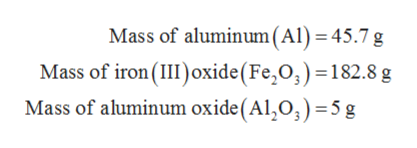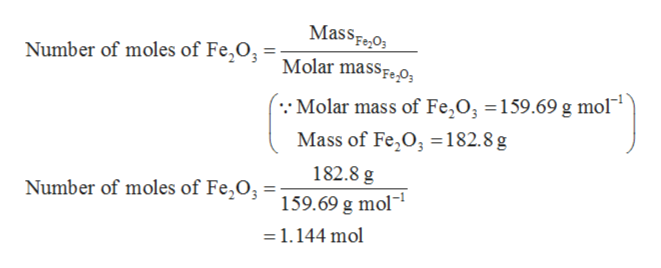# In one experiment you reacted 45.7g of Aluminum with 182.8g of Iron (III) oxide to produce Aluminum Oxide and Elemental iron (not diatomic).a. How many mols of Iron (III) oxide do you have?b. Which is the limiting agent?c. If you produced 5 grams of aluminum oxide, how many atoms of aluminum are in 5 grams?

Question
30 views

In one experiment you reacted 45.7g of Aluminum with 182.8g of Iron (III) oxide to produce Aluminum Oxide and Elemental iron (not diatomic).

a. How many mols of Iron (III) oxide do you have?

b. Which is the limiting agent?

c. If you produced 5 grams of aluminum oxide, how many atoms of aluminum are in 5 grams?

check_circle

Step 1

The molecules that present inside any substance can be determined by calculating the number of moles. These moles are then multiplied by the Avogadro’s number to get the molecules present in the substance.

Step 2

Given data:help_outlineImage TranscriptioncloseMass of aluminum (Al) = 45.7 g Mass of iron (II oxide (Fe,O,) -182.8 g Mass of aluminum oxide(Al,0,) =5 g fullscreen
Step 3

Part (a)

The number of moles of iron (III) oxide (Fe2O3) is ca...help_outlineImage TranscriptioncloseMassFeO Molar masseO Number of moles of Fe2O Molar mass of Fe,O; = 159.69 g mol1 Mass of Fe,O, 182.8g 182.8 g Number of moles of Fe,O 159.69 g mol =1.144 mol fullscreen

### Want to see the full answer?

See Solution

#### Want to see this answer and more?

Solutions are written by subject experts who are available 24/7. Questions are typically answered within 1 hour.*

See Solution
*Response times may vary by subject and question.
Tagged in

### Inorganic Chemistry